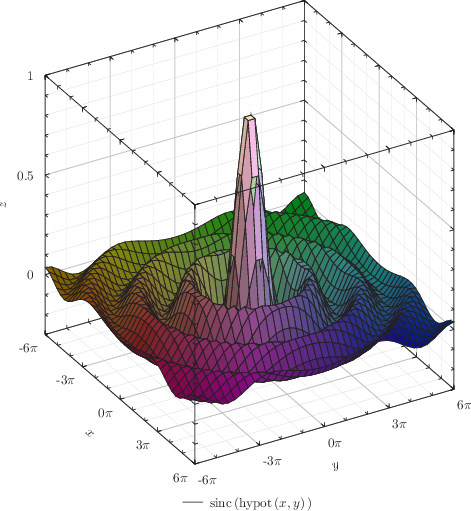## 8.14.1 Surface plotting

The surface plot style is similar to the colormap and contourmap plot styles, but produces maps of the valuesof functions of two variables using three-dimensional surfaces. The surface is displayed as a grid of four-sided elements, whose number may be specified using the set samples command, as in the example

set samples grid 40x40


If data is supplied from a data file, then it is first re-sampled onto a regular grid using one of the methods described in Section 8.12.

The example below plots a surface indicating the magnitude of the imaginary part of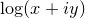:

set numerics complex
set xlabel r"Re($z$)"
set ylabel r"Im($z$)"
set zlabel r"$mathrm{Im}(mathrm{log}[z])$"
set key below
set size 8 square
set grid
set view -30,30
plot 3d [-10:10][-10:10] Im(log(x+i*y))with surface col black fillcol blue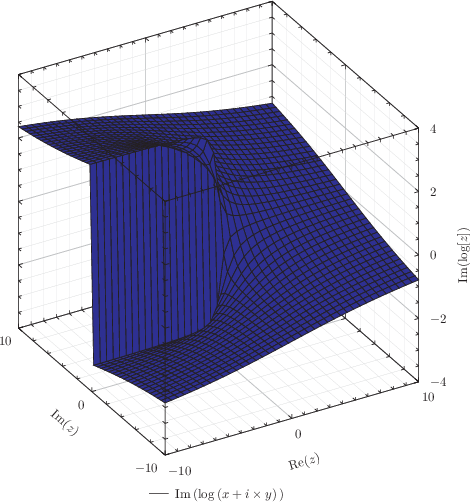Example: A surface plotted above a contour map

In this example, we plot a surface showing the value of the expression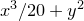, and project below it a series of contours in the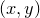plane.
set nokey
set size 8 square
plot 3d x**3/20+y**2 with surface col black fillc red,x**3/20+y**2 with contours col black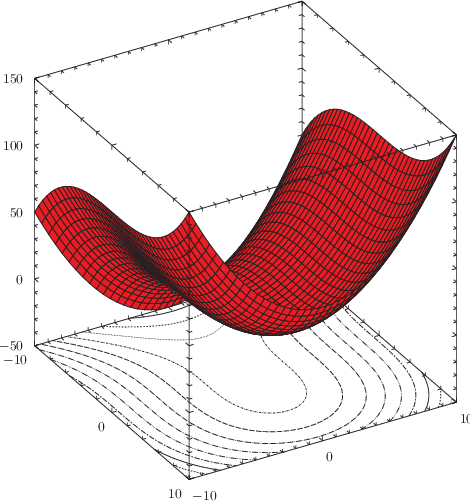Example: The sinc(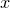) function represented as a surface

In this example, we produce a surface showing the functionwhere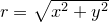. To produce a prettier result, we vary the color of the surface such that the hue of the surface varies with azimuthal position, its saturation varies with radius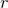, and its brightness varies with height.
set numerics complex
set xlabel "$x$"
set ylabel "$y$"
set zlabel "$z$"
set xformat r"%s$pi$"%(x/pi)
set yformat r"%s$pi$"%(y/pi)
set xtics 3*pi ; set mxtics pi
set ytics 3*pi ; set mytics pi
set ztics
set key below
set size 8 square
set grid
plot 3d [-6*pi:6*pi][-6*pi:6*pi][-0.3:1] sinc(hypot(x,y))with surface col blackfillcol hsb(atan2($1,$2)/(2*pi)+0.5,hypot($1,$2)/30+0.2,\$3*0.5+0.5)